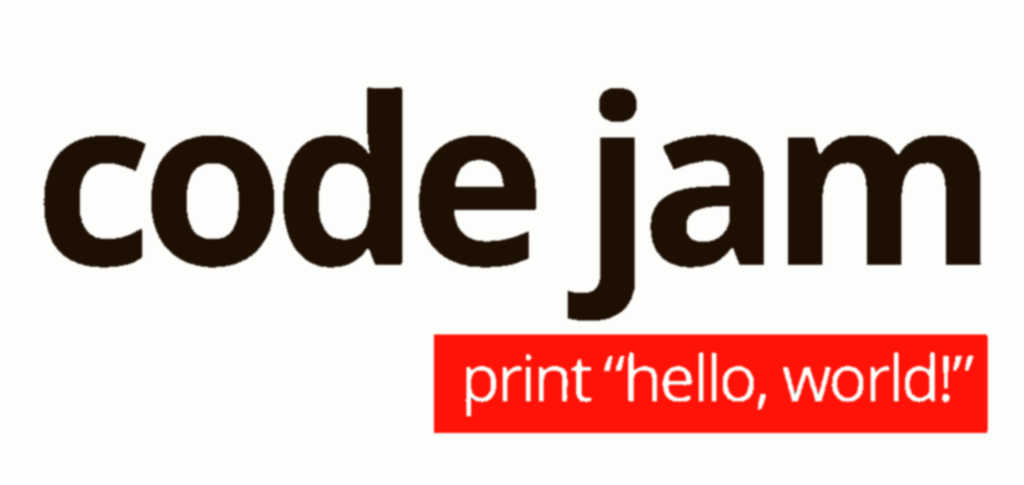Moons and Umbrellas2021-03-30T12:34:26+02:00
Code Jam gcj2021 Haskell

Problem B of this year’s qualifier is a simple “greedy-DP” puzzle. We’re given a chain of a binary string with holes, and must determine some cost function’s optimal value over the possible ways to fill it up.

It’s almost as easy to solve as the statement’s length makes it seem, so I’ll try and find something to spice it up.

First off, the input string is given as a dumbed-down ASCII representation of Cody-Jamal’s true Artwork. This can be fixed with a simple stream transcoder:

``````fixASCII ∷ String → String
fixASCII = map \$ \case 'C' → '🌘'
'J' → '🌂'
'?' → '❓'
x   →  x``````

Now for each prefix of the input mural, we’ll want to know the minimal fee for that part of the mural, so we can deduce the minimal fee after adding a glyph to it, and reap the final fee in the end.

But that won’t work: there’s no way to achieve the “deduction” part. It can be fudged to work, though. We can split the minimal fee information in two: the minimal fee assuming the prefix ends in a moon, and the minimal fee assuming the prefix ends in an umbrella.

The natural way to represent this is with a simple record:

``data State = State { (🌘) ∷ Maybe Int, (🌂) ∷ Maybe Int }``

Each `Int` represents the minimal cost of a mural ending in said glyph; `Nothing` if it’s impossible to end as such. But that’s not perfect yet. Such a representation allows for a pair of `Nothing`s, and… what would we answer if we ended on such a pair? Because of the transition function’s structure, such a pair can never occur. So we really need a representation that reflects that.

According to the kind folks on IRC1, the standard library to reach for in this case is `these`.

Except the Haskell setup we’ve got over at GCJ’s is even more primeval than CodinGame’s!2 So no libraries for us. Luckily enough, the data type in itself is pretty straightforward.

``data These a b = This a | That b | These a b``

Err… since I’m not using the library, I might as well give those constructors meaningful names.

``data (│) = (:🌘) Int | (:🌂) Int | (:🌘🌂) Int Int``

Much better. I won’t reproduce its standard combinators and `Bifunctor` instances here, they’re just a reproduction of `These`’s.

With the helpers available, here’s the little utility to compute the final minimal cost from the split state.

``````minimal ∷ (│) → Int
minimal = merge min``````

It allows for a delightfully elegant implementation of the transition function.

``````
transition ∷ Int → Int → Char → (│) → (│)
transition x y = \case '🌘' →         (:🌘)   .  (<|🌘)
'🌂' →         (:🌂)   .             (<|🌂)
'❓' → uncurry (:🌘🌂) . ((<|🌘) &&& (<|🌂))
where (<|🌘) = minimal . (🌂) (+y)
(<|🌂) = minimal . (🌘) (+x)``````

Maybe inserting a small reading key would be in order? So, assuming we could implement things properly using the `these` package, here’s what the various symbols mean:

• The type name is `(│)`, aiming to evoke horizontal separation, as it is most often used to represent a state of the computation between two mural glyphs.
• `(:🌘)`, `(:🌂)`, `(:🌘🌂)` are `This`, `That` and `These`, the data constructors holding integer values associated to a moon, an umbrella or both, respectively.3
• `(🌘)` and `(🌂)` are `first` and `second` from `Bifunctor`: apply a function to the moon or umbrella aspect of the value.
• `(<|🌘)` and `(<|🌂)` are helpers to perform the “append moon” and “append umbrella” operations.

We need dedicated code to create the initial state, as there’s no proto-state that would result in the correct first one if the transition function were blindly applied to it.

``````start ∷ Char → (│)
start '🌘' = (:🌘) 0
start '🌂' = (:🌂) 0
start '❓' = (:🌘🌂) 0 0``````

And now solving the problem reduces to a simple fold.

``````invoice ∷ Int → Int → String → Int
invoice x y (h:t) = minimal \$ foldl' (flip (transition x y)) (start h) t``````

It performs linearly, which is optimal in this case, and solves test sets 1, 2 and 3 without a hitch.

This concludes this qualifier problem B’s solution. The complete code with I/O handling is on my github4.

See you soon for problem D!

1. Thank you, @Guillaum and @lyxia!↩︎

2. And that’s saying something.↩︎

3. Prefixing an emoji with a colon makes it a capital Emoji, AKA a constructor in Haskell parlance.↩︎

4. Set the theme to night mode. It’s the only correct way to render a moon!↩︎